# Examples On Addition And Subtraction Of Vectors

Go back to  'Vectors and 3-D Geometry'

Example – 1

From any two vectors $$\vec a\,\,{\rm{and}}\,\,\vec b$$ , prove that

(i) $$\left| {\,\vec a + \vec b\,} \right| \le \left| {\,\vec a\,} \right| + \left| {\,\vec b\,} \right|$$

(ii) $$\left| {\,\vec a - \vec b\,} \right| \le \left| {\,\vec a\,} \right| + \left| {\,\vec b\,} \right|$$

(iii) $$\left| {\,\vec a + \vec b\,} \right| \ge \left| {\left| {\,\vec a\,} \right| - \left| {\,\vec b\,} \right|} \right|$$

When does the equality hold in these cases?

Solution: Consider this figure: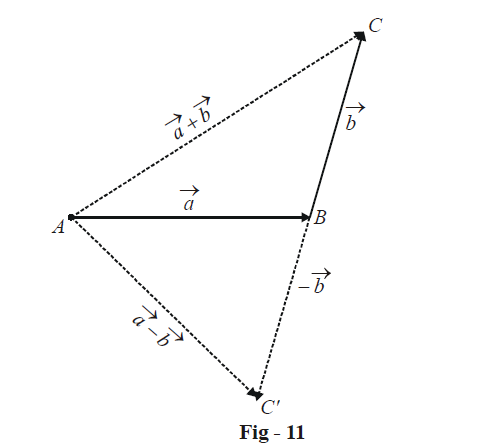The first two relations follow from the fact that in any triangle, the sum of two sides is greater than the third side:

In $$\Delta$$ ABC:                   $$AC \le AB + BC$$(we’ll soon talk about how and when the equality comes)

$$\Rightarrow \qquad \quad \left| {\vec a + \vec b} \right| \le \left| {\vec a} \right| + \left| {\vec b} \right|$$

In $$\Delta$$ ABC ':                $$AC' \le AB + BC' = AB + BC$$

$$\Rightarrow \qquad \quad \left| {\vec a - \vec b} \right| \le \left| {\vec a} \right| + \left| {\vec b} \right|$$

In the first relation, the equality can hold only if the two vectors have the same direction; this should be intuitively obvious: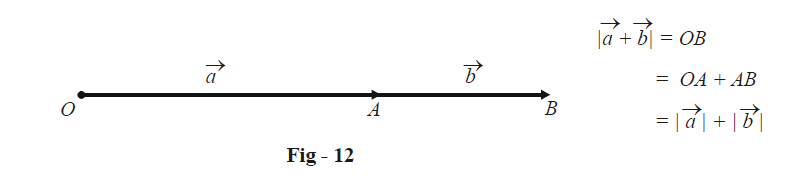The equality in the second relation holds if the two vectors are exactly opposite: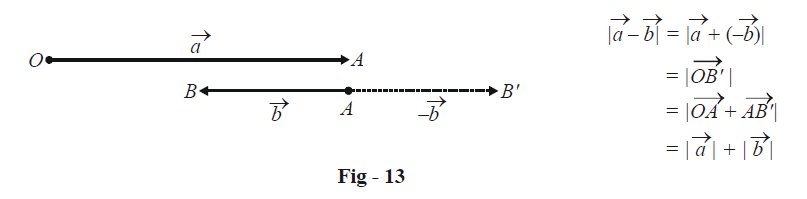To prove the third relation, we use in $$\Delta$$ABC in Fig - 11, the geometrical fact that the difference of any two sides of a triangle is less than its third side:

$\qquad\left| {AB - BC} \right| \le AC$

$\Rightarrow \quad \left| {\left| {\vec a} \right| - \left| {\vec b} \right|} \right| \le \left| {\vec a + \vec b} \right|$

The equality holds when $$\vec a\,\,{\rm{and}}\,\,\vec b$$ are precisely in the opposite direction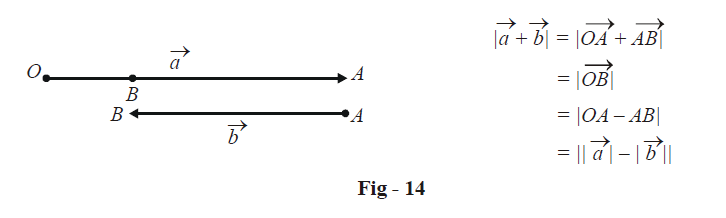The main point to understand from this example is how easily vector relations follows from corresponding geometrical facts.

Example – 2

Suppose that the vectors $$\vec a\,\,{\rm{and}}\,\,\vec b$$ represent two adjacent sides of a regular hexagon. Find the vectors representing the other sides.

Solution: Let the hexagon be A1A2A3A4A5A6, as shown: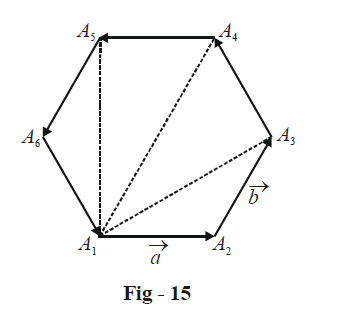First of all, we note an important geometrical property of a regular hexagon:

\begin{align}&\quad{Diagonal{\rm{ }}\qquad =\qquad {\rm{ }}2{\rm{ }} \times {\rm{ }}side}\\&{ \Rightarrow \quad\;\; {A_1}{A_4}{\rm{ }}\qquad =\qquad {\rm{ }}2{\rm{ }} \times {A_2}{A_3}}\end{align}

Also, since  $${A_1}{A_{4}}||{A_2}{A_3}$$, we have

\begin{align}\quad\overrightarrow {{A_1}{A_4}}\qquad&\rm{ =\qquad 2} \times \overrightarrow {{A_2}{A_3}} \\&=\qquad 2\,\vec b\end{align}

Now we use the triangle law to determine the various sides:

\begin{align}\;\;\overrightarrow {{A_3}{A_4}}\quad & =\quad \overrightarrow {{A_1}{A_4}} - \overrightarrow {{A_1}{A_3}} \\\;\;\;\quad\qquad&=\quad 2\vec b - \left( {\vec a + \vec b} \right)\\\qquad\quad\;\;\;&=\quad\vec b - \vec a\\\;\;\; \overrightarrow {{A_4}{A_5}}\quad &=\;\;\;\; - \vec a\left( {only{\rm{ \;}}the{\rm{\; }}sense{\rm{\; }}differs;{\rm{\;}}support{\rm{ \;}}is{\rm{\; }}parallel{\rm{ \;}}to{\rm{\; }}the{\rm{\;}}support{\rm{ \;}}of{\rm{\; }}\vec a{\rm{ }}} \right)\\\;\;\;\overrightarrow {{A_5}{A_6}}\quad &=\quad - \,\overrightarrow {{A_2}{A_3}} \\\qquad\quad &= \quad - \vec b\\~~\overrightarrow {{A_6}{A_1}}\quad & = \quad - \,\overrightarrow {{A_3}{A_4}} \\ \qquad~~~&= \quad\vec a - \vec b\end{align}

Thus, all sides are expressible in terms of $$\vec a\,\,{\rm{and}}\,\,\vec b$$.

Example – 3

What can be interpreted about  $$\vec a\,\,{\rm{and}}\,\,\vec b$$ if they satisfy the relation:

$\left| {\vec a + \vec b} \right| = \left| {\vec a - \vec b} \right|$

Solution: Make  $$\vec a\,\,{\rm{and}}\,\,\vec b$$  co-initial so that they form the adjacent sides of a parallelogram: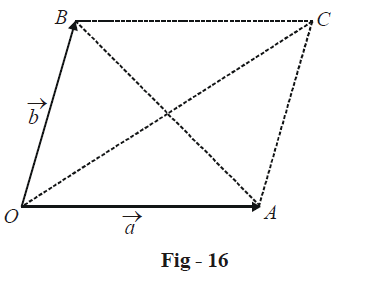We have,

$\left| {\vec a + \vec b} \right| = \left| {\overrightarrow {OC} } \right| = OC$

$and\;\left| {\vec a - \vec b} \right| = \left| {\overrightarrow {BA} } \right| = BA$

Thus, the stated relation implies that the two diagonals of the parallelogram OACB are equal, which can only happen if OACB is a rectangle.

This implies that $$\vec a\,\,{\rm{and}}\,\,\vec b$$ form the adjacent sides of a rectangle. In other words, $$\vec a\,\,{\rm{and}}\,\,\vec b$$ are perpendicular to each other.

Vectors
grade 11 | Questions Set 1
Vectors